Setting the learning objective profile target is step 9 of the tutorial: how do I create a course that adapts to my learners. It is advisable to first go through the tutorial before setting the learning profile target.

Learning objectives are inseparably related to Learning profile targets. In a learning profile target you can weigh the learning objectives. Within an adaptive block activities which will best help achieve the learning profile target will be offered to the learner. So if a learner doesn't score that well on a learning objective, the platform will offer an activity which pays the most attention to that learning objective.

Only if you have added all adaptive blocks and filled the activities therein with questions linked to learning objectives, you can start setting the learning profile target. You will have to set this after you have added all questions, because the values you will add here will shift if you add questions to learning objectives later on.

Let's go back to the learning objectives-tab.

In the overview below you see that values are filled in under the group 'Grammar'.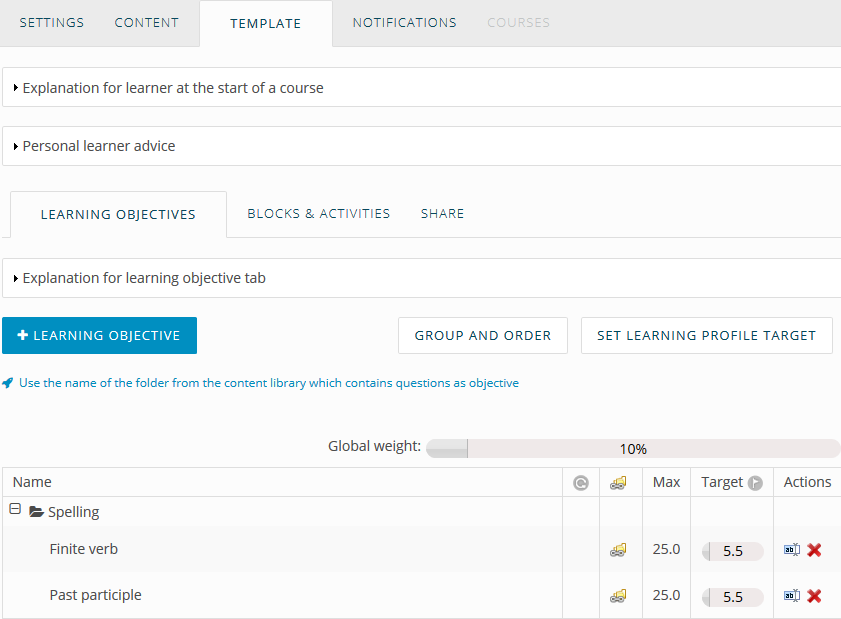First a quick explanation of the different columns:

####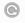MemoTrainer

Tells you if there are questions linked to that learning objective in the MemoTrainer. In the example above this is not the case, yet. We will do this in step 11.

####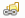Copied learning objective

Tells if the name of the learning objectives is copied from the content. Because we have done this in step 4, this icon is shown.

#### Max

The maximum amount of points that can be achieved in this learning objective. Because a questions is always worth 1 point, this number equals the amount of questions linked to the learning objective.

#### Target

The amount a learner has to score in order to reach 100% on the learning objective. The global weight is standardly set on 80%, but this is not always desirable. We will give this part extra attention now. Click on Set learning profile target. You will see the following screen: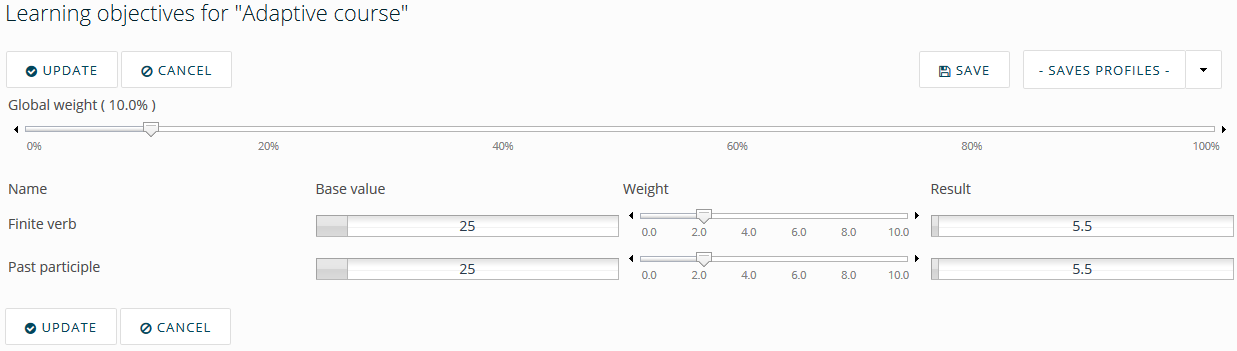#### Base value

The same as 'Max' in the previous screen. The maximum amount of points that can be accumulated for this learning objective. Because a question equals 1 point, this number represents all questions linked to that learning objective.

#### Result

The same as 'Target' in the previous screen. The amount of points that have to be accumulated in order to complete the learning objective.

#### Weight

With the weight you can influence the result. First, set the global weight to 10%, this will allow to set the weight more accurately.

A bit more explanation about the scoring:

In the activities where learning objectives are linked to questions, the learner will receive one point (+1) per correctly answered question. When a question is answered incorrectly, a point will be reducted (-1). If you have determined (in step 5) that learners will have to have 5 questions answered correctly in order to skip the learning objective, the result should be set to 5 as well.

#### Example:

In the example below the pretest has been taken. For the questions that are linked to Finite verb, 5 questions (x1 point) have been answered correctly. This means they have reached the target of 5 points and therefore have completed the learning objective. A total of 3 questions have been answered correctly and 2 questions incorrectly for Past participle. This translates to a total of 3 points minus 2 points equals 1 (3-2=1). A total of 1 point has been given to this learning objective, Past participle.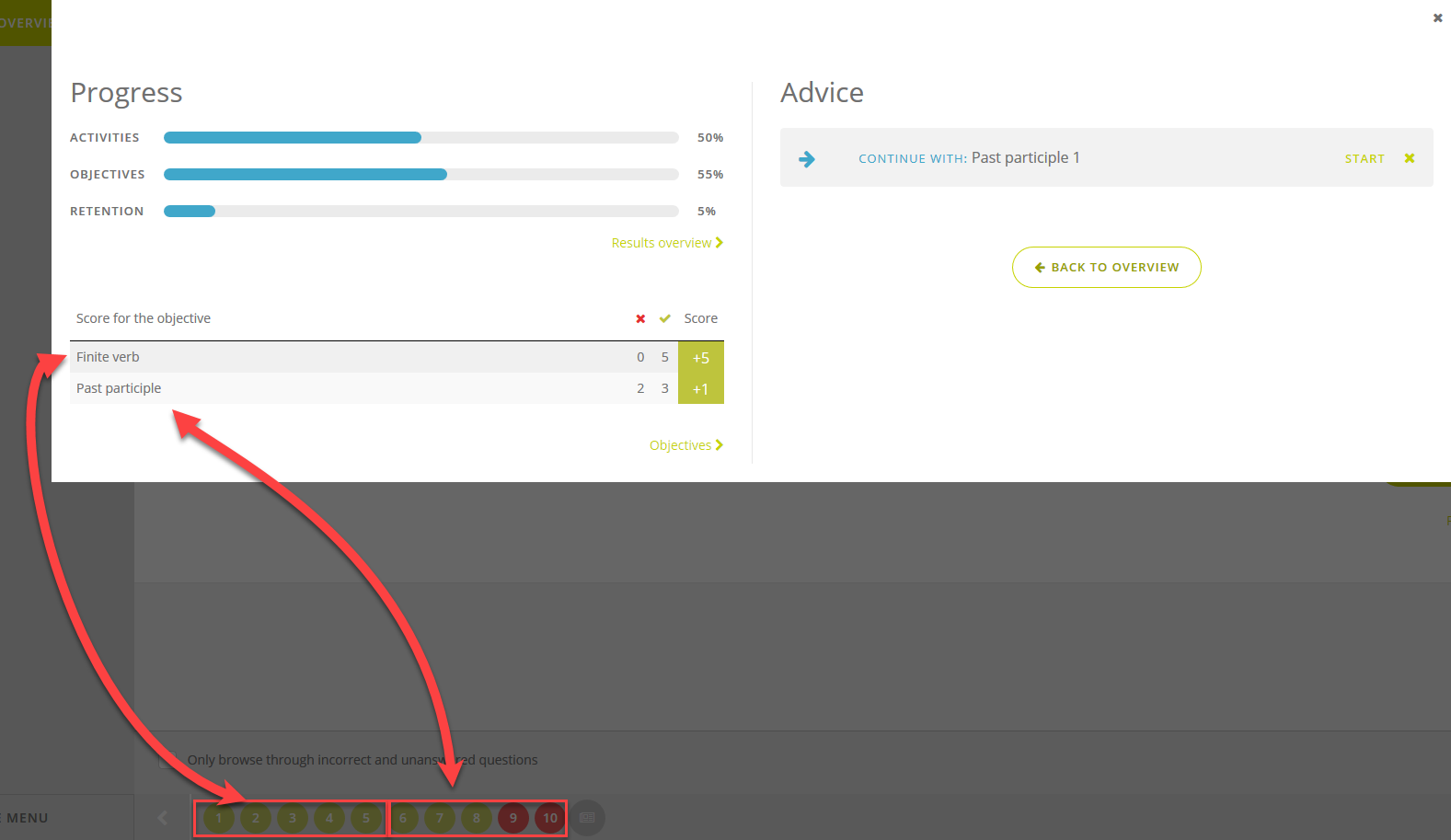The consequence of these results are that there will be no lessons available for Finite verb, because the target of the learning objective has been met. For the learning objective Past participle, this means that there will be lessons available in which the learners can accumulate more points to reach their goal; 1 point has been achieved, 4 points to go: (The learning objective Finite verb still can be trained via the MemoTrainer, which we will configure in step 11)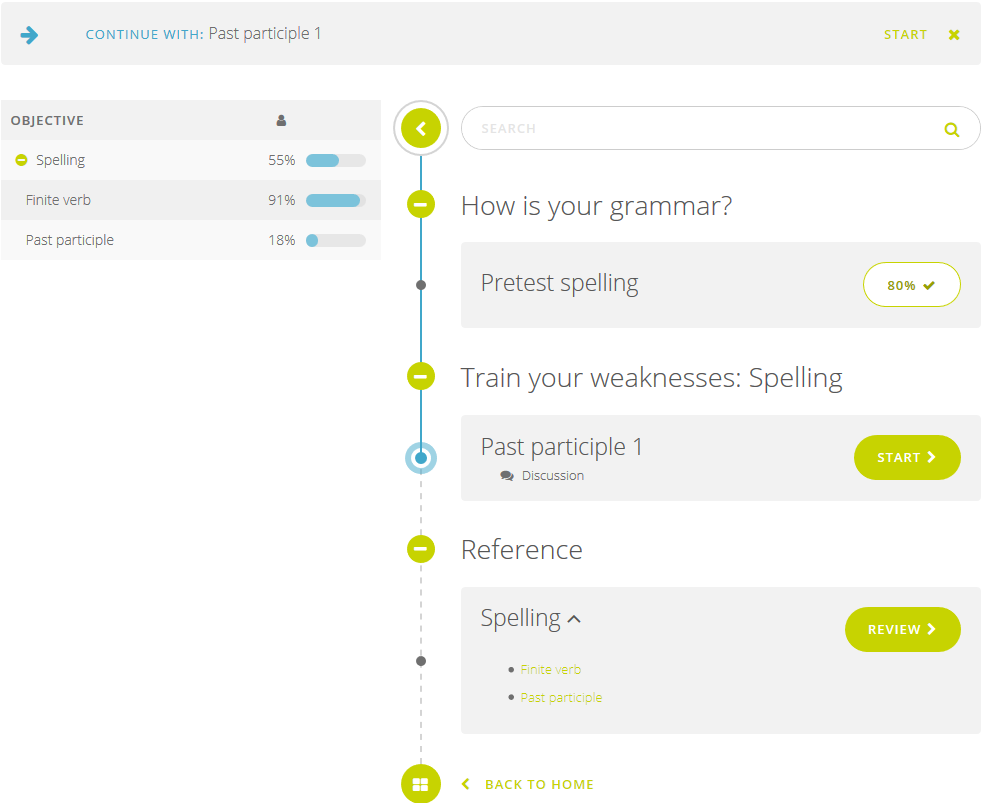The activity 'Past participle 1' comes from the adaptive block we have created in step 7. In that activity 5 new questions are linked to the learning objective Past participle. In the example above another 4 points have to be accumulated. The question is: what are the possible scenario's to achieve this?

 Number of correct questions Number of incorrect questions Amount of points after this activity Final score for 'Past participle' Amount of points to accumulate 5 0 5 5 Learning objective completed! 4 1 3 (4-1) 4 1 3 2 1 (3-2) 2 3 2 3 -1 (2-3) 0 5 1 4 -2 (1-4) 0 5

Conclusion: only if all questions have been answered correctly, no lessons about this learning objective will be available. The set result of '5' in the learning objective target may seem little, but when you take a closer look at the scoring it isn't so bad because the learning objectives are created quite specific.

Pay attention when setting the learning profile target that the target isn't set to high. There must be enough lessons to come available so that the learner can reach the target.